### Textures, Motion, Derivatives

The following table is an extension to the previous. These variables give information about the intersection point for texture mapping. They are defined when the ray has hit a textured object:

 type name content miVector * tex_list list of texture coordinates miVector * bump_x_list list of X bump basis vectors miVector * bump_y_list list of Y bump basis vectors miVector tex texture coord (tex shaders) miVector motion interpolated motion vector miVector * derivs list of surface derivatives

tex_list

A pointer to an array containing the texture coordinates of the intersection point in all texture spaces. When material shaders that support multiple textures on a surface call a texture lookup function, they must specify which texture coordinate to use, usually by choosing one of the texture vertices in tex_list and copying it to tex. In mental ray 3.4 texture spaces in miBox may have arbitrary dimension. tex_list entries contain only coordinates from up to three dimensional texture spaces. For higher dimensions, tex_list entries are set to zero and the function mi_texture_interpolate must be used instead.

bump_x_list

A pointer to an array containing the x bump basis vectors in all texture spaces. The vectors are in object space. The scene must have been prepared to contain or compute these vectors. They are very rarely used because normally shader compute the basis vectors on the fly.

bump_y_list

A pointer to an array containing the y bump basis vectors in all texture spaces. The vectors are in object space.

tex

The texture coordinates where the texture should be evaluated. This variable is available only in texture shaders; it is set by the caller (for example, a texture lookup from a material shader).

motion

The motion vector of the intersection point. If the object has no motion vectors ( m statements in the vertices in the scene description file) or inherited motion transformations (including camera motion), the motion vector is a null vector. mental ray versions before 3.2 compute the motion vector only if there is a nonzero shutter interval; mental ray 3.2 and later always interpolate motion vectors if present. However, even mental ray 3.2 does not interpolate motion transformations if motion blurring is turned off, either with a zero shutter interval or a motion off statement in the options block, because of the performance implications.

derivs[ ]

A pointer to an array containing the surface derivative vectors. If the object is a free-form surface object, the surface derivative vectors derivsderivs contain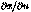,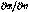,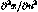,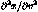,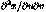, respectively. Heredenotes the intersection point. For polygonal objects the surface derivatives are taken from the .mi file. If the object has no first derivative vectors, the first two vectors are null vectors. If the object has no second derivative vectors, the last three vectors are null vectors. Since surface derivatives are calculated approximately, the vectors,and the normal vector at the intersection point are not necessarily orthogonal to each other.

Copyright © 1986-2007 by mental images GmbH